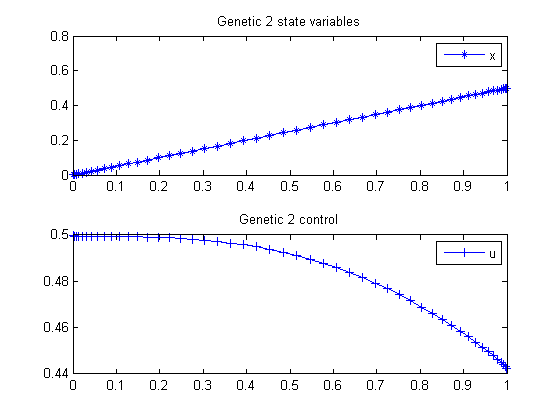41  Genetic 2

PROCEEDINGS OF WORLD ACADEMY OF SCIENCE, ENGINEERING AND TECHNOLOGY VOLUME 21 JANUARY 2007 ISSN 1307-6884

Optimal Control Problem, Quasi-Assignment Problem and Genetic Algorithm Omid S. Fard and Akbar H. Borzabadi

See paper for failure of GA toolbox algorithm.

Example 2

41.1  Problem Formulation

Find u over t in [0; 1 ] to minimize

J =
 1 0
u2 dt

subject to:

 dx dt
=
 1 2
*x2*sin(x) + u

The initial condition are:

 x(0) = 0
 x(1) = 0.5

Reference: 

41.2  Problem setup

toms t
p = tomPhase('p', t, 0, 1, 50);
setPhase(p);

tomStates x
tomControls u

% Initial guess
x0 = {icollocate(x == 0.5*t)
collocate(u == 0)};

% Boundary constraints
cbnd = {initial(x == 0)
final(x == 0.5)};

% ODEs and path constraints
ceq = collocate(dot(x) == 1/2*x.^2.*sin(x)+u);

% Objective
objective = integrate(u.^2);

41.3  Solve the problem

options = struct;
options.name = 'Genetic 2';
solution = ezsolve(objective, {cbnd, ceq}, x0, options);
t = subs(collocate(t),solution);
x = subs(collocate(x),solution);
u = subs(collocate(u),solution);
Problem type appears to be: qpcon
Starting numeric solver
===== * * * =================================================================== * * *
TOMLAB - Tomlab Optimization Inc. Development license  999001. Valid to 2011-02-05
=====================================================================================
Problem: ---  1: Genetic 2                      f_k       0.235327080033222360
sum(|constr|)      0.000000001551798634
f(x_k) + sum(|constr|)      0.235327081585021000
f(x_0)      0.000000000000000000

Solver: snopt.  EXIT=0.  INFORM=1.
SNOPT 7.2-5 NLP code
Optimality conditions satisfied

FuncEv    1 ConstrEv   23 ConJacEv   23 Iter   21 MinorIter   71
CPU time: 0.093750 sec. Elapsed time: 0.093000 sec.

41.4  Plot result

subplot(2,1,1)
plot(t,x,'*-');
legend('x');
title('Genetic 2 state variables');

subplot(2,1,2)
plot(t,u,'+-');
legend('u');
title('Genetic 2 control');